Publication date: 08/13/2020

## Contributions

#### T2

The T2 contributions for a PCA or PLS model with p variables and k components are calculated as: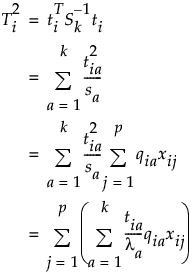where

ti = the vector of k scores for the ith observation

Sk = the diagonal sample covariance matrix of the k scores for historical observations. For PCA models, Sk is the diagonal eigenvalue matrix.

sa = the ath diagonal element of Sk

rja = the jth element of the ath eigenvector for PCA models and the ath column of the Rk loading matrix for PLS models. Rk is the matrix used to relate the score matrix, Tk to the X matrix, such that Tk=XRk.

xij = the value of the jth variable for the ith observation.

Note: The p terms in the last sum are the variable contributions.

The contribution of each variable is the sum of its contribution to each score, weighted by the normalized score value. A variable is considered to have a large contribution to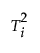if there is a large normalized score value, and the variable contribution is large.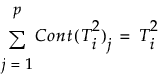the contribution proportion of variable j is defined as: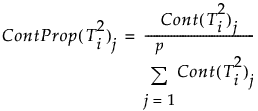Note: When computing T2 contribution proportions, JMP zeros out negative contributions. Negative contributions are possible due to the interaction of variables during the projection of X in PCA and PLS models. The negative contributions are zeroed in order to identify the variable contributions that represent a large proportion of the total positive contributions.

#### DModX

For PCA and PLS models, the contribution of variable j to DModXi is defined as: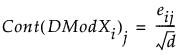Note that since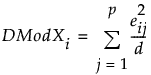the contribution proportion of variable j is defined as: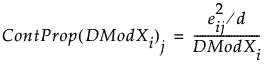#### SPE

For PCA and PLS models, the contribution of variable j to SPEi is defined as: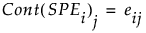Note that since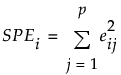the contribution proportion of variable j is defined as: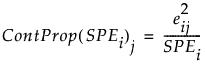#### Score Contributions

The score contribution computation is the same as T2 contributions but are computed only for the dimensions selected in the score plot.